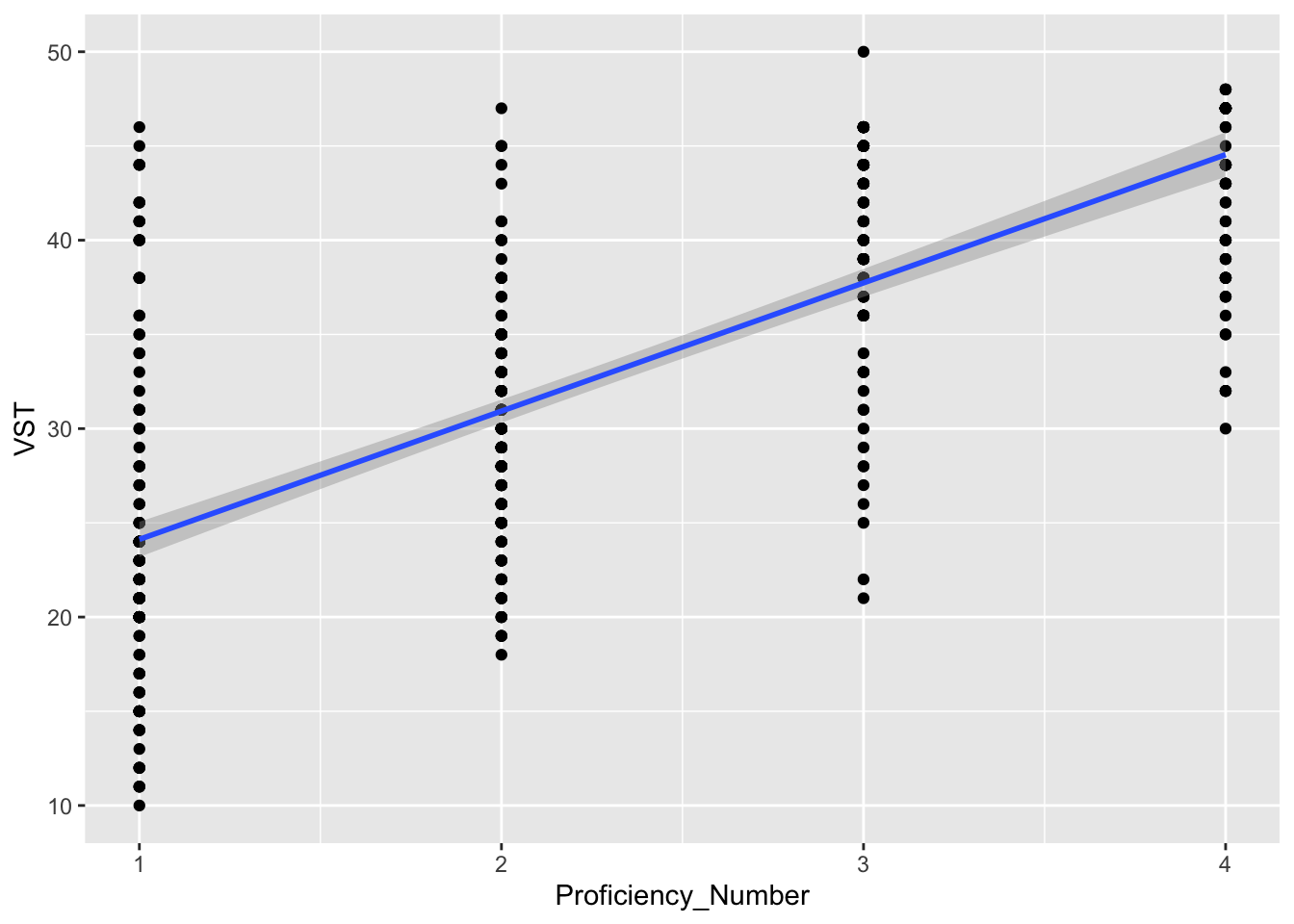In previous tutorials, we have used dataframes that are part of the R base package or are part of ggplot2. Here, we will load data from a spreadsheet that is formatted as a comma separated values (.csv) document.

Our first step will be to open a new R script and save it in a convenient location (e.g., in a folder named “Rday3” on your desktop).

Our second step will be to download the target .csv file, extract it, and save a copy of the target .csv file (“ICNALE_500_simple.csv”) in a folder called “data” in the Rday3 folder.

Third, with our new R script open in RStudio, we will click on “Session” in the toolbar and “Set Working Directory”" to “Source File Location”. This will let RStudio know where to look for your file(s).

Finally, we will load our target .csv file (“ICNALE_500_simple.csv”) as a dataframe (and in this case we will call that dataframe “fundata”). NOTE- click on the “code” button to see the code used in each section. For this page, the default is for the code to be hidden - try to write the code on your own before checking the answer.

``````fundata <- read.csv("data/ICNALE_500_simple.csv", header = TRUE) #this presumes that the .csv file is in a subfolder entitled "data"
summary(fundata)``````
``````##   Participant   Nationality       VST        Proficiency_CEFR
##  CHN_001:  1   JPN    :139   Min.   :10.00   A2_0:146
##  CHN_010:  1   THA    :130   1st Qu.:24.00   B1_1:159
##  CHN_012:  1   SIN    : 67   Median :33.00   B1_2:124
##  CHN_013:  1   CHN    : 63   Mean   :32.56   B2_0: 71
##  CHN_014:  1   KOR    : 54   3rd Qu.:41.00
##  CHN_016:  1   TWN    : 25   Max.   :50.00
##  (Other):494   (Other): 22
##  Proficiency_Number
##  Min.   :1.00
##  1st Qu.:1.00
##  Median :2.00
##  Mean   :2.24
##  3rd Qu.:3.00
##  Max.   :4.00
## ``````

Based on the summary output, we see that our dataframe has four variables (Participant, Nationality, VST, Proficiency_CEFR, and Proficiency_Number).

These variable names represent the following: - Participant: Participant identifier - Nationality: Nationality of participant - VST: Score on receptive vocabulary size test - Proficiency_CEFR: Categorical proficiency rating based on the Common European Framework of Reference for Languges (CEFR) - Proficiency_Number: CEFR rating converted to a numerical scale (ranging from 1 to 4)

### Visualizing the data

Lets apply what we learned in the last tutorial to this new data. Don’t forget to load ggplot2!

``library(ggplot2)``

#### Scatterplots

First, lets create a scatterplot with a line of best fit to examine the relationship between VST (y-axis) and Proficiency_Number (x-axis). What does the scatterplot indicate about the relationship between VST scores and Proficiency?

``````ggplot(data = fundata) + #this line indicates my dataset
geom_point(mapping = aes(x = Proficiency_Number, y = VST)) + #this line sets the x and y axis
geom_smooth(mapping = aes(x = Proficiency_Number, y = VST), method = lm)``````Now try to make the following plot, which shows the data by nationality (hint, I also used geom_jitter()).

``````ggplot(data = fundata) + #this line indicates my dataset
geom_jitter(mapping = aes(x = Proficiency_Number, y = VST, color = Nationality)) + #this line sets the x and y axis
geom_smooth(mapping = aes(x = Proficiency_Number, y = VST), method = lm)``````